# GED Math : Perimeter and Sides

## Example Questions

← Previous 1

### Example Question #1 : Other Shapes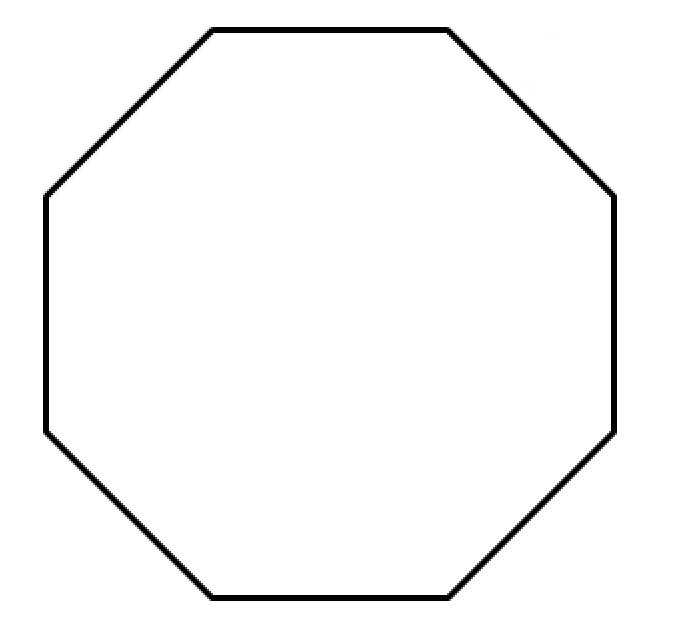Identify the above polygon.

Octagon

Pentagon

Hexagon

Octagon

Explanation:

A polygon with eight sides is called an octagon.

### Example Question #2 : Other Shapes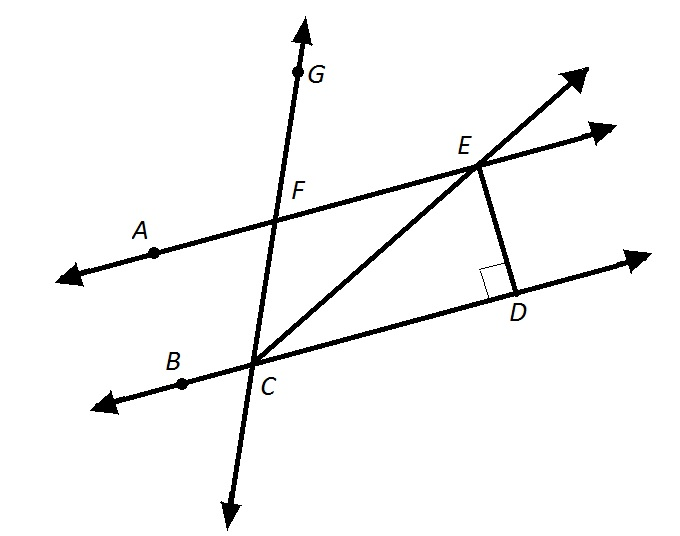Refer to the above figure.

Which of the following is not a valid alternative name for Polygon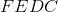?

Polygon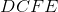Polygon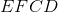Polygon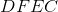Polygon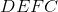PolygonExplanation:

In naming a polygon, the vertices must be written in the order in which they are positioned, going either clockwise or counterclockwise. Of the four choices, only Polygonviolates this convention, sinceandare not adjacent vertices (nor are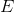and).

### Example Question #1 : Perimeter And Sides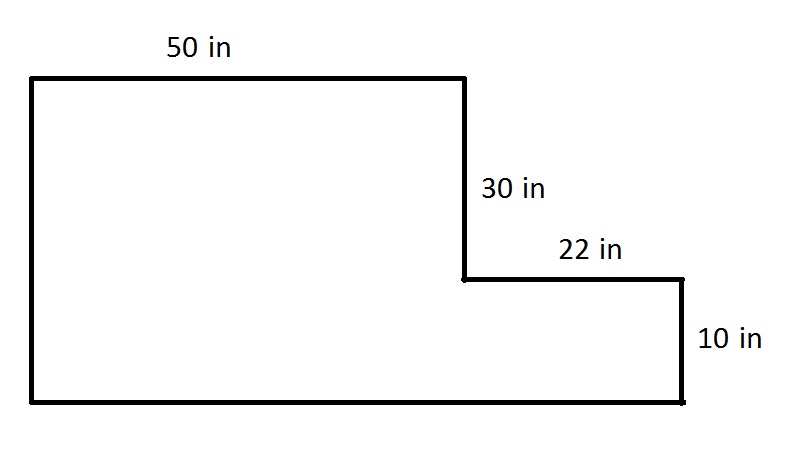Refer to the above figure. All angles shown are right angles.

What is the perimeter of the figure?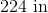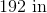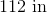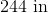Explanation:

The figure can be viewed as the composite of rectangles. As such, we can take advantage of the fact that opposite sides of a rectangle have the same length, as follows: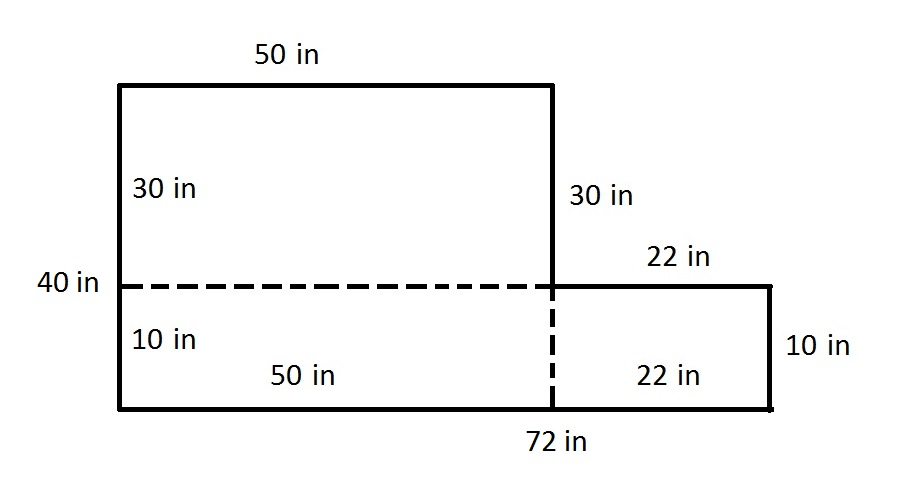Now that the missing sidelengths are known, we can add the sidelengths to find the perimeter: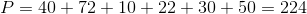### Example Question #4 : Other Shapes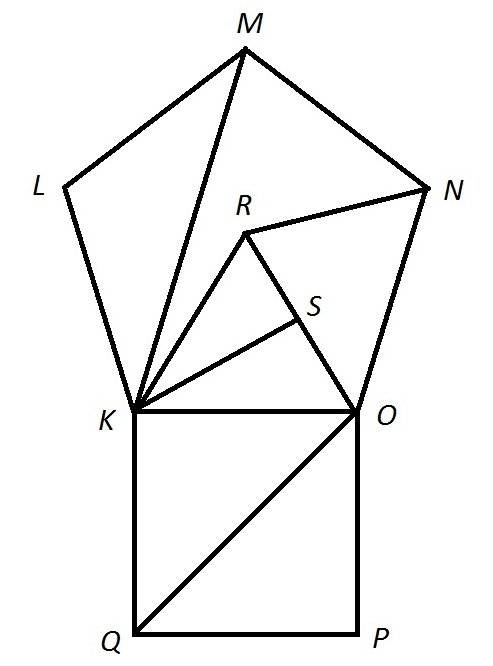Refer to the above figure.

Which of the following segments is a diagonal of Pentagon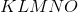?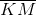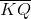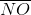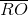Explanation:

A diagonal of a polygon is a segment whose endpoints are nonconsecutive vertices of the polygon. Of the four choices, onlyfits this description.

### Example Question #5 : Other Shapes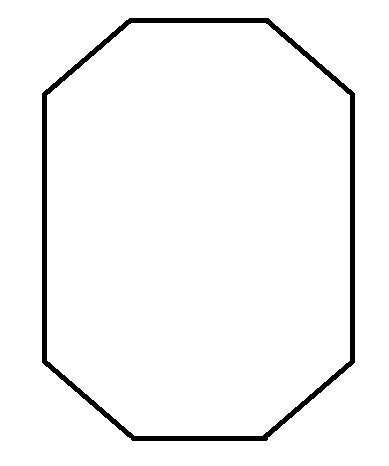Classify the above polygon.

Octagon

Pentagon

Hexagon

Rhombus

Octagon

Explanation:

A polygon with eight sides is called an octagon.

### Example Question #6 : Other Shapes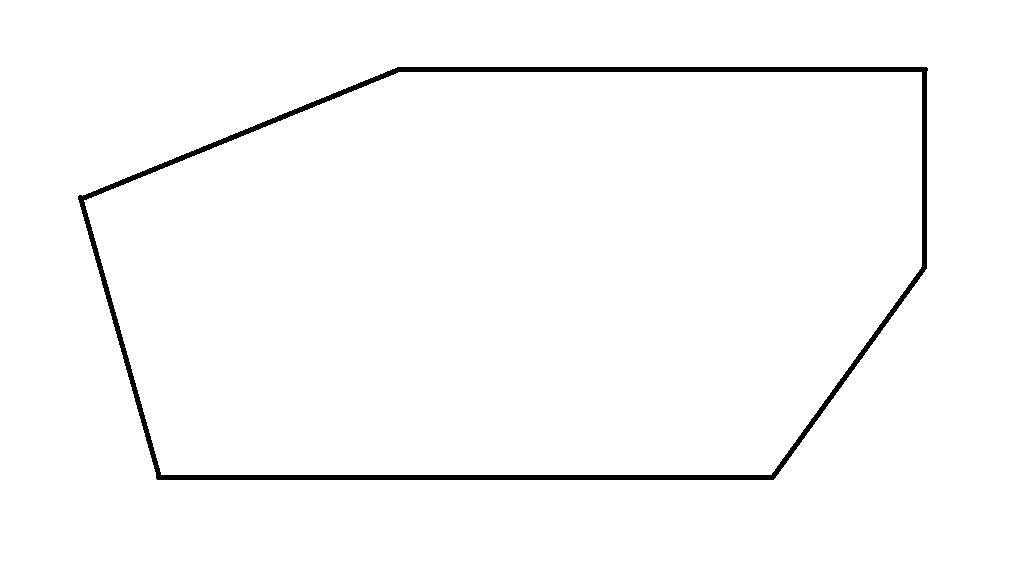Classify the above polygon.

Hexagon

Trapezoid

Pentagon

Octagon

Hexagon

Explanation:

A polygon with six sides is called a hexagon.

### Example Question #2 : Other Shapes

Hexagon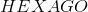is regular. If diagonals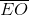and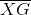are constructed, which of the following classifications applies to Quadrilateral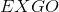?

I) Rectangle

II) Rhombus

III) Square

IV) Trapezoid

IV only

I only

II only

I, II, and III only

I only

Explanation:

The figure described is below.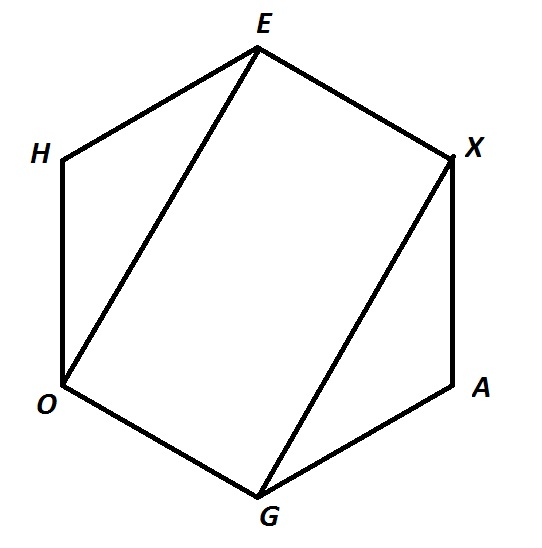Since the hexagon is regular, its sides are congruent, and its angles each have measure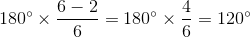.

Also, each of the triangles are isosceles, and their acute angles measure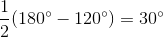each. This means that each of the four angles of Quadrilateralmeasures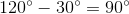, so Quadrilateralis a rectangle. However, not all sides are congruent, so it is not a rhombus. Also, since it is a rectangle, it cannot be a trapezoid.

The correct response is I only.

### Example Question #8 : Other Shapes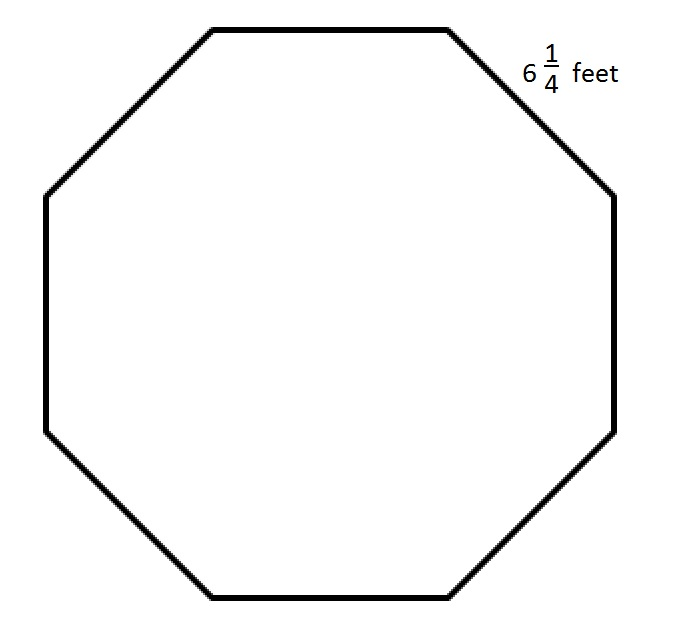The above figure is a regular octagon. Give its perimeter in yards.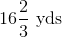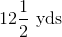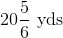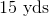Explanation:

A regular octagon has eight sides of equal length, so multiply the length of one side by eight: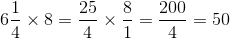feet.

Divide by three to get the equivalent in yards: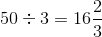yards.

### Example Question #9 : Other Shapes

What is the perimeter of a semicircle with an area of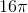?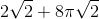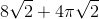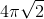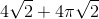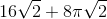Explanation:

Write the formula for the area of a semicircle.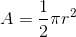Substitute the area.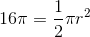Multiply by 2, and divide by pi on both sides.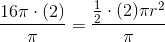The equation becomes: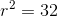Square root both sides and factor the right side.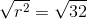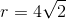The diameter is double the radius.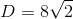The circumference is half the circumference of a full circle.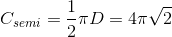The perimeter is the sum of the diameter and the half circumference.

The answer is:### Example Question #10 : Other Shapes

A hexagon has a perimeter of 90in.  Find the length of one side.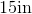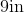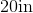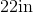Explanation:

A hexagon has 6 equal sides. The formula to find perimeter of a hexagon is: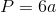where a is the length of any side. Now, to find the length of one side, we will solve for a

We know the perimeter of the hexagon is 90in. So, we will substitute and solve for a. We get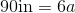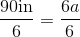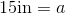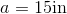Therefore, the length of one side of the hexagon is 15in.

← Previous 1